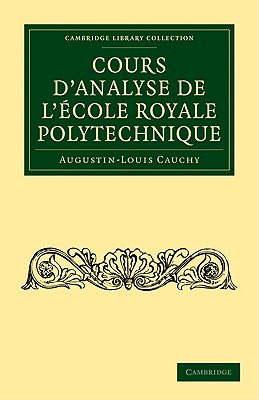Cours d'Analyse de l'École Royale Polytechnique (Cambridge Library Collection - Mathematics)

Paperback

List Price: 82.00*
* Individual store prices may vary.

Description

In 1821, the French mathematician Augustin-Louis Cauchy published Cours d'Analyse de L' cole Royale Polytechnique, a textbook designed to teach his students the basic theorems of calculus in as rigorous a way as possible. Cauchy was a pioneer of mathematical analysis, a branch of mathematics concerned with the idea of a limit, whether of a sequence or of a function. This book consists of 12 chapters that discuss real functions, infinitely small and large quantities, substitution groups, symmetrical functions, unknown variables, imaginary functions, and rational fractions in a recurrent series. It also provides formulas for solving various problems, such as converting the sine and cosine of a multiple polynomial arc and the Lagrange interpolation. Cauchy built on the work of Leibniz and Newton and is generally regarded as one of the greatest mathematicians in history. This is a reissue of one of his most important contributions.

Cambridge University Press, 9781108002080, 604pp.

Publication Date: July 20, 2009